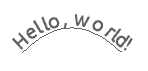NAME

Prima::CurvedText - fit text to path

DESCRIPTION

The module registers single function `curved_text_out` in `Prima::Drawable` namespace. The function plots the line of text along the path, which given as a set of points. Various options regulate behavior of the function when glyphs collide with the path boundaries and each other.

SYNOPSIS

``````  use Prima qw(Application Drawable::CurvedText);
\$spline = [qw(100 100 150 150 200 100)];
\$::application-> begin_paint;
\$::application-> spline(\$spline);
\$::application-> curved_text_out( 'Hello, world!', \$::application-> render_spline( \$spline ));``````curved_text_out \$TEXT, \$POLYLINE, %OPTIONS

`\$TEXT` is a line of text, no special treatment is given to tab and newline characters. The text is plotted over `\$POLYLINE` path that should be an array of coordinate numeric pairs, in the same format as `Prima::Drawable::polyline` expects.

The text begins to plot by drawing the first glyphs at the first path point, unless specified otherwise with the `offset` option. The glyph is plotted with the angle perpendicular to the path segment; therefore the path may contain floating point numbers if futher plotting angle accuracy is desired.

When text cannot be fit along a single segment, it is plotted along the next segment in the path. Depending on the `bevel` boolean option, the next glyph is either simply drawn on the next segment with the angle corresponding to the tangent of that segment (value 0), or is drawn with the normal text concatenation offset, with the angle averaged between tangents of the two segments it is plotted between (value 1). The default value of `bevel` option is 1.

The glyph positioning rules differ depending on `collisions` integer option. If 0 (default), the next glyph position always corresponds with the glyph width as projected to the path. That means, that glyphs will overlap when plotted inside segments forming an acute angle. Also, when plotting along a reflex angle, the glyphs will be visually more distant from each other that when plotted along the straight line.

Simple collision detection can be turned on with setting `collisions` to 1 so that no two neighbour glyphs may overlap. Also, the glyphs will be moved together to the minimal distance, when possible. With this option set the function will behave slower. If detection of not only neighbouring glyphs is required, `collisions` value can be set to 2, in which case a glyph is guaranteedly will never overlap any other glyph. This option may be needed when, for example, text is plotted inside an acute angle and upper parts of glyphs plotted along one segment will overlap with lower parts of glyphs plotted along the other one. Setting `collisions` to 2 will slow the function even more.

The function internally creates an array of tuples where each contains text, plotting angle, and horisontal and vertical coordinates for the text to be plotted. In the array context the function returns this array. In the scalar context the function returns the success flag that is the result of last call to `text_out`.

Options:

bevel BOOLEAN=true

If set, glyphs between two adjoining segments will be plotted with bevelled angle. Otherwise glyphs will strictly follow the angles of the segments in the path.

callback CODE(\$SELF, \$POLYLINE, \$CHUNKS)

If set, the callback is called with `\$CHUNKS` after the calculations were made but before the text is plotted. `\$CHUNKS` is an array of tuples where each consists of text, angle, x and y coordinates for each text. The callback is free to modify the array.

collisions INTEGER=0

If 0, collision detection is disabled, glyphs plotted along the path. If 1, no two neighbour glyphs may overlap, and no two neighbour glyph will be situated further away from each other than it is necessary. If 2, same functionality as with 1, and also two glyphs (in all text) will overlap.

nodraw BOOLEAN=false

If set, calculate glyph positions but do not draw them.

offset INTEGER=0

Sets offset from the beginning of the path where the first glyph is plotted. If offset is negative, it is calculated from the end of the path.

skiptail BOOLEAN=false

If set, the remainder of the text that is left after the path is completely traversed, is not shown. Otherwise (default), the tail text is shown with the angle used to plot the last glyph (if bevelling was requested) or the angle perpendicular to the last path segment (otherwise).

AUTHOR

Dmitry Karasik, <dmitry@karasik.eu.org>.